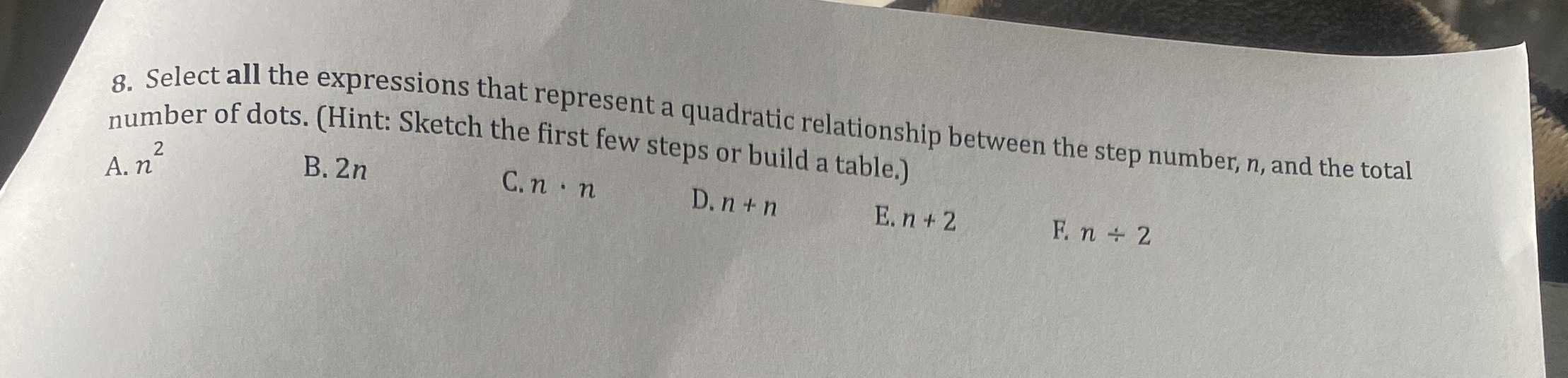### ¿Todavía tienes preguntas de matemáticas?

Pregunte a nuestros tutores expertos
Algebra
Pregunta8. Select all the expressions that represent a quadratic relationship between the step number, $$n$$ , and the total number of dots. (Hint: Sketch the first few steps or build a table.)

A. $$n ^ { 2 }$$ B. $$2 n$$ C. $$n \cdot n n$$ D. $$n + n$$ E. $$n + 2$$ F. $$n \div 2$$

A)$$n^2$$
C)$$n*n$$